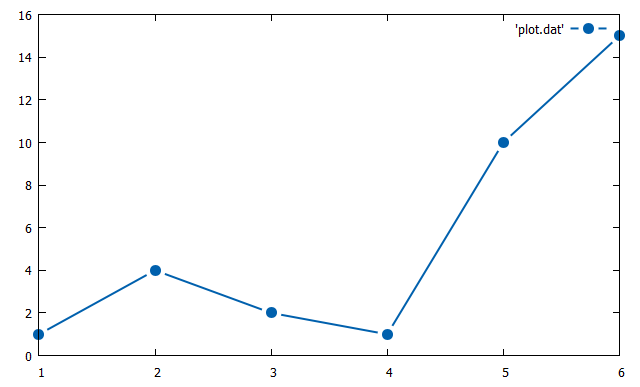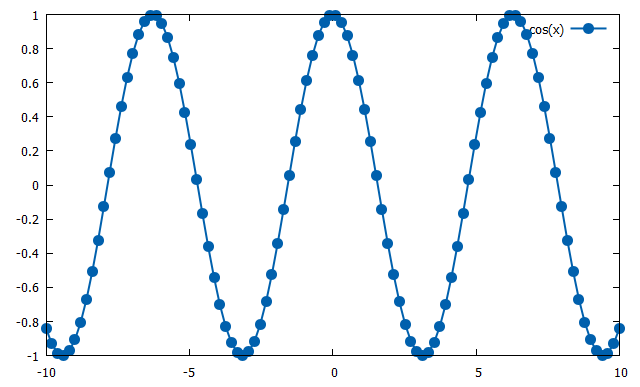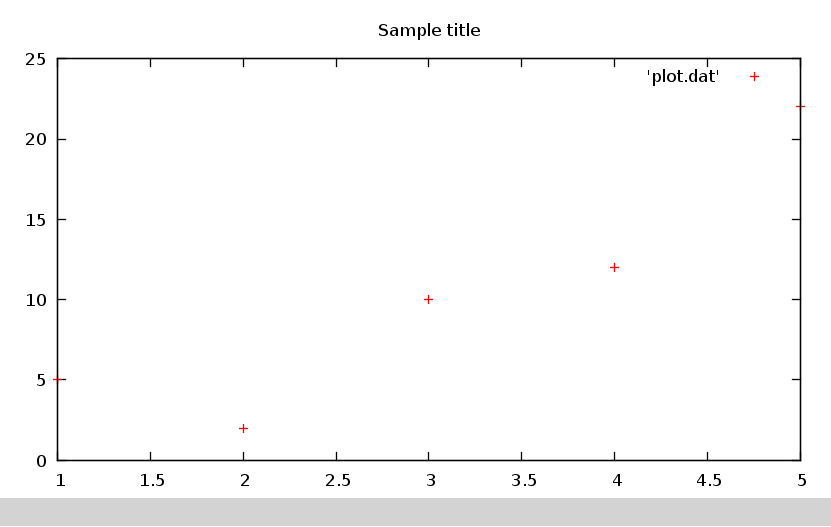Plotvar : The developer corner - GNUplot

Generate a line plot with GNUplot

set style line 1 lc rgb '#0060ad' lt 1 lw 2 pt 7 pi -1 ps 1.5
set pointintervalbox 2
plot 'plot.dat' with linespoints ls 1

Assuming you put this kind of data in plot.dat (use pwd in GNUplot to see where to put this file).

# plotting_data1.dat
# X   Y
1   1
2   4
3   2
4   1
5   10
6   15

You will get :GNUplot line cos plot

Let's start another test with cosinus.
We keep style and line (between points).

set style line 1 lc rgb '#0060ad' lt 1 lw 2 pt 7 pi -1 ps 1.5
set pointintervalbox 0
plot cos(x) with linespoints ls 1

This simply print cos values with default sample rate.

Who tells GNUplot is hard to use?

Let's see the result :C code creates a graph automatically using GNUplot

To have a window which opens when you launch the C programs you need to install gnuplot-x11 (or some other gnuplot ui).

Thanks to Maberib for this post.

#include
#include
#define NUM_POINTS 5
#define NUM_COMMANDS 2

int main()
{
char * commandsForGnuplot[] = {"set title 'Sample title'", "plot 'plot.dat'"};
double xvals[NUM_POINTS] = {1.0, 2.0, 3.0, 4.0, 5.0};
double yvals[NUM_POINTS] = {5.0 ,3.0, 10.0, 3.0, 22.0};
FILE * temp = fopen("plot.dat", "w");
FILE * gnuplotPipe = popen ("gnuplot -persistent", "w");
int i;
for (i=0; i < NUM_POINTS; i++)
{
fprintf(temp, "%lf %lf \n", xvals[i], yvals[i]);
}

for (i=0; i < NUM_COMMANDS; i++)
{
fprintf(gnuplotPipe, "%s \n", commandsForGnuplot[i]);
}
return 0;
}

This will print your plot into gnuplot user interface.

See what we get :